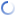MSTE
Office for Mathematics, Science, and Technology Education
College of Education @ University of Illinois

# Relative Prime Probability

This applet is designed to choose random integers and check whether they are relatively prime. This demonstrates the theorem that the probability of two positive integers chosen at random being relatively prime is six divided by pi squared.

6/π2 ≈ 0.6079271018540266

## Experiment

MeasurementValue
# Trials0
# Relatively Prime0
Ratio (Prime / Total)0
π ≈ √6 / Ratio0

### Generated Integer Pairs